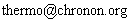## Coupled pendulums applet

This applet was written to investigate how energy is dispersed from one large object into many smaller objects. There is a massive pendulum which is coupled to 119 lighter pendulums.

 You do not appear to have Java enabled on this browser. (Note that Java is no longer supplied by default on Windows systems). The latest Java environment can be downloaded and installed from the Java website

### Description of the program

We take L to be the length of the large pendulum, and M its mass. Θ is the angle with the upward vertical. The generalized momentum corresponding to Θ is P = ML².Θ

In the program there are two indices i and j ranging from 0..16 and 0..6 respectively, but below these are represented by a single index y (or z) which is thought of as ranging over all of the smaller pendulums. These have have unit length and mass and angle θy with the upward vertical, so the generalized momentum is just .θy

The coupling between each small pendulum and its four neighbours is k and that between the large pendulum and each small one is k2.

The Hamiltonian of the system is then:
H = ½ML².Θ ² + MLg(1 + sin Θ) +
 Σ y
[ ½.θy² + k2(1 − cos(Θy) ) + g(1 + sin θy)] +
k (1 − cos(θy − θz) )
hence we get the equations of motion:
..Θ =
 .P ML²
= − g cosθ/L −
 Σ y
k2 sin(Θ −θy)/ML²
and
..θy = .py = −g cos θy/L + k2 sin(Θ − θy) −
The idea behind this applet was to demonstrate the ideas of second law of thermodynamics. I had thought that the energy of the large pendulum would quickly be transferred to the smaller pendulums, or even if the transfer was slow then it would be fairly steady. However this doesn't happen. Energy is transferred to the smaller pendulums but then is transferred back again. I am intending to look into what it is in the nature of this system that makes things happen this way. Any suggestions for further reading will be gratefully received. Contact: